#Interactive Real Analysis

Next | Previous | Glossary | Map

## Cauchy Condensation Test

### Examples 4.2.8:

Use the Cauchy Condensation criteria to answer the following questions:
1. In the sum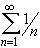, list the terms a 4, a k, and a 2 k. Then show that this series (called the harmonic series) diverges.
2. For which p does the series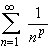converge or diverge ?

The sequence { 1/n } corresponds to the harmonic series. Therefore:

• a 4 = 1/4
• a k = 1/k
• a 2 k = 1 / 2 k
As for convergence or divergence of this series, we already know, by 'elementary' means, that this series diverges. Here is an alternative proof of this, using the Cauchy Condensation test as follows: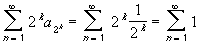and the last series diverges by the Divergence test. Hence, the original series also diverges.

Next, we investigate the seriesfor various p:

• If p < 0 then the sequence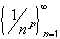converges to infinity. Hence, the series diverges by the Divergence Test.
• If p > 0 then consider the series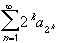=The right hand series is now a Geometric Series, so that:
• if 0 < p1 then 2 1-p1, hence the right-hand series diverges
• if 1 < p then 2 1-p < 1, hence the right-hand series converges
But this is exactly what we need, in conjunction with Cauchy's Condensation test, to finish the proof of the statement.
Next | Previous | Glossary | Map